# Math classification

In 3A class are 27 students. One-third got a B in math, and the rest got an A. How many students received a B in math?

n =  9

### Step-by-step explanation:

$n=27\mathrm{/}3=9$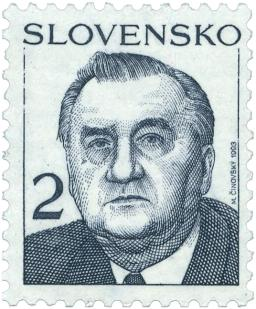Did you find an error or inaccuracy? Feel free to write us. Thank you!## Related math problems and questions:

• School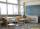27 students of 6.D class are going on a trip. They pay 9 € each. The teacher got 153 €. How many children have not paid?
• Math test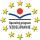In class 6.A are 26 students, and the teacher had managed to give tests to 13 students. 13 testes he gave in 6.5 min. How many pupils still haven't test? How long will it take the teacher to give tests to 43 students?
• Students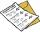The class has 22 students. 2 of which have assessments of 2. The count of assessment of 1 is three times more than the count of students with an assessment of 3. How many students have an assessment of 1, and how many students have an assessment of 1.
• Warehouses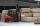To four warehouses is going cement in 25 kg bags. To first one third, to second quarter of the total. The third store got two thirds of the rest, and the last 310 tons came to fourth. How many cement is in all warehouses and how much got every one?
• TrioTrio of workers earn 750 euros. Money divided by the work that each of them made​​. First received twice as the second, the second received three times more than the third. How many got everyone from workers?
• News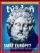From 28 students buy magazine 10 The economist students, 10 Pravda students and 18 students don't buy any of these magazines. How many students buy both magazines?
• English and math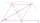1/5 of a class study English, and 3/5 study mathematics. If the class consist of 80 students, how many students study English and mathematics in the class?
• DivisionWhich number in division 16 give 12 and the rest 3?
• Class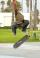The class has 18 students. Everyone knows inline skating or skateboarding. Inline skating can ride 11 students on a skateboard 10. How many ride on inline skates and on skateboard?
• Lunch timeIn a cafeteria, 3/10 of the students are eating salads, and 3/5 are eating sandwiches. There are 30 students in the cafeteria. How many students are eating lunches other than salads or sandwiches?
• Class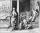When Pythagoras asked how many students attend the school, he said: "Half of the students studying mathematics, 1/4 music, seventh silent, and there are three girls at school." How many students had Pythagoras at school?
• Beet campaign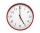The beet's field is being harvested. Beets are transported to 3 plants, 3 trucks. The first car returns to the field 3 times an hour, the second car after 30 minutes, and the third car 5 times an hour. All cars left at 8 in the morning. How many cars do w
• Cents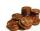Julka has 3 cents more than Hugo. Together they have 27 cents. How many cents has Julka and how many Hugo?
• Class alphabet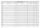All 29 pupils in the class are written in a class by alphabet. The number of pupils enrolled before Paul is three times higher than the number of pupils behind him. Calculate how many pupils are enrolled after Paul.
• Expression plus minusEvaluate expression: (-1)2 . 12 – 6 : 3 + (-3) . (-2) + 22 – (-3) . 2
• FractionsSort fractions z1 = (6)/(11); z2 = (10)/(21); z3 = (19)/(22) by its size. Result write as three serial numbers 1,2,3.
• 3 cats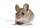3 cats eat 3 mice in 3 days. How many mice will be eaten by 10 cats in 10 days?